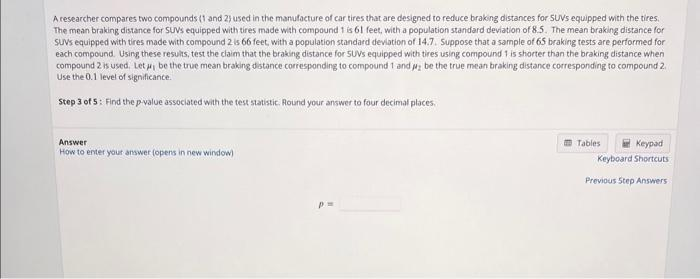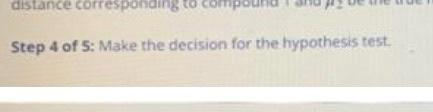# (Solved): will upvote if correct please help A researcher compares two compounds 1 and 2 f used in the manufac ...A researcher compares two compounds 1 and 2 f used in the manufacture of car tires that are designed to reduce brakig distances for $$5 U N$$ s equipped with the tires. The mean braking distance for SUNs equipped with tires made with compound 1 is 61 feet, with a population standard devlation of $$8.5$$. The mean braking distance for SUNs equipped with tires made with compound 2 is 66 feet, with a population standard deviation of $$14.7$$. 5 uppose that a sample of 65 braking tests are pertormed for each compound. Using these resulis, test the claim that the braking distance for 5 UVs equipped with tires using compound 1 is shorter than the braking distance when compound 2 is used; Let $$\mu_{1}$$ be the true mean braking distance corresponding to compound 1 and $$\mu_{2}$$ be the true mean braking distance corresponding to campound 2 . Use the $$0.1$$ level of significance. Step 3 of 5 : Find the p-yalue associated with the test statistic. Hoind your answer to four decimal places. Answer How to enter yout answer (opens in new window) Keyboard Shortcuts Step 5 of 5: State the conclusion of the hypothesis test. Step 4 of 5: Make the decision for the hypothesis test.

We have an Answer from Expert